# 1.构建框架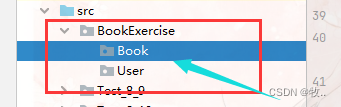## 1.1Book 底下的 图书 类 和 书架类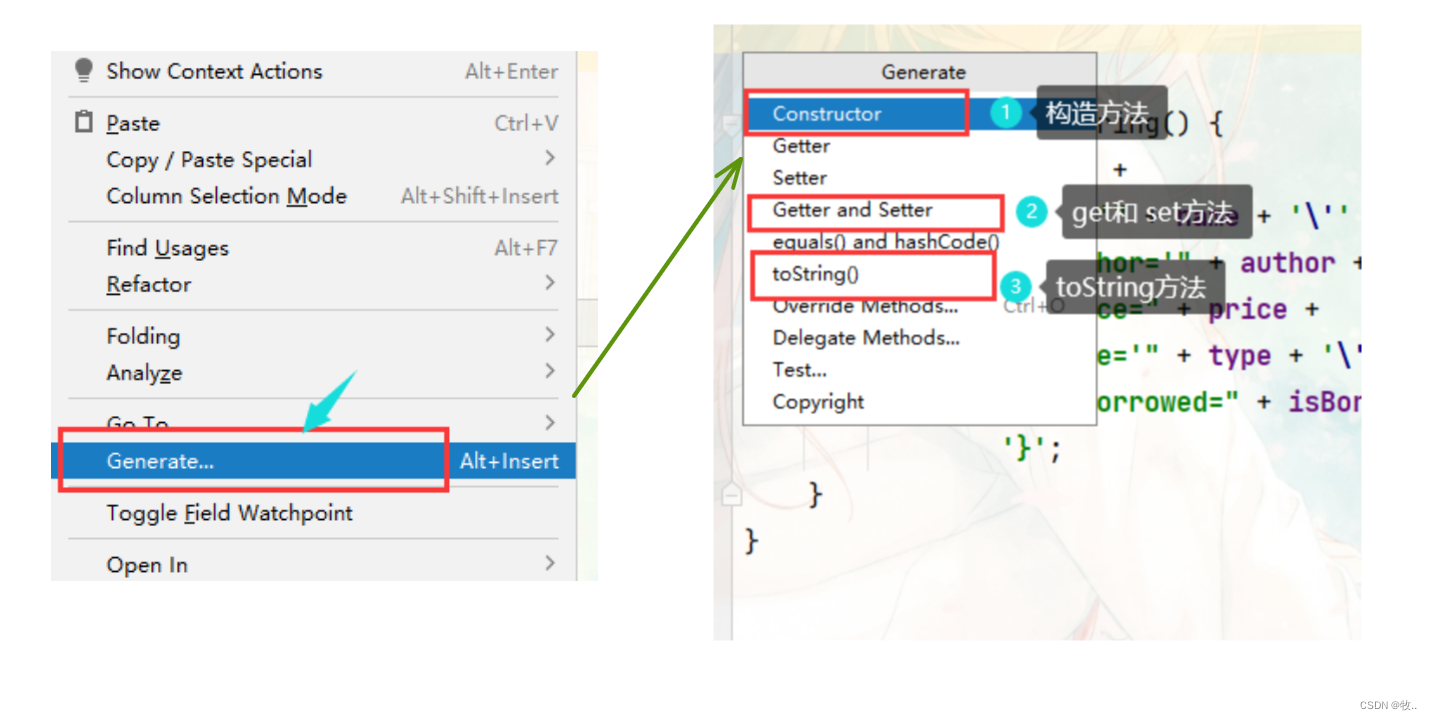``````Book 类

public class Book {

private  String name; // 书名
private  String author; // 作者
private int price; // 价格
private String type; // 类型
private boolean isBorrowed;// 是否借出
// 这个 可以不用 写进 toString, new 一个 isBorrowed 默认没有借出

给定 普通成员变量。 封装起来 给定 特有 的get 和 set 方法

// 构造方法

public Book(String name, String author, int price, String type) {
this.name = name;
this.author = author;
this.price = price;
this.type = type;
}

public String getName() {
return name;
}

public void setName(String name) {
this.name = name;
}

public String getAuthor() {
return author;
}

public void setAuthor(String author) {
this.author = author;
}

public int getPrice() {
return price;
}

public void setPrice(int price) {
this.price = price;
}

public String getType() {
return type;
}

public void setType(String type) {
this.type = type;
}

public boolean isBorrowed() {
return isBorrowed;
}

public void setBorrowed(boolean borrowed) {
isBorrowed = borrowed;
}

@Override
public String toString() {
return "Book{" +
"name='" + name + '\'' +
", author='" + author + '\'' +
", price=" + price +
", type='" + type + '\'' +
", isBorrowed=" + isBorrowed +
'}';
}
}
``````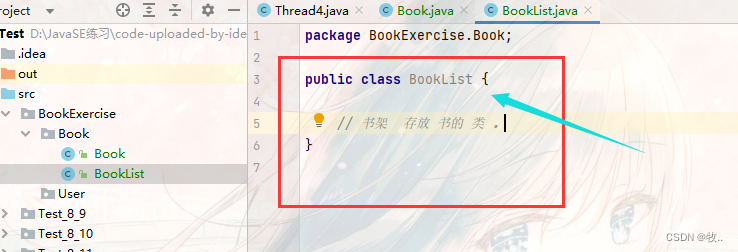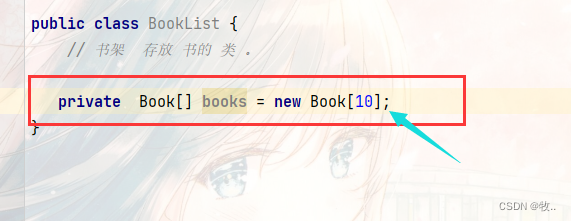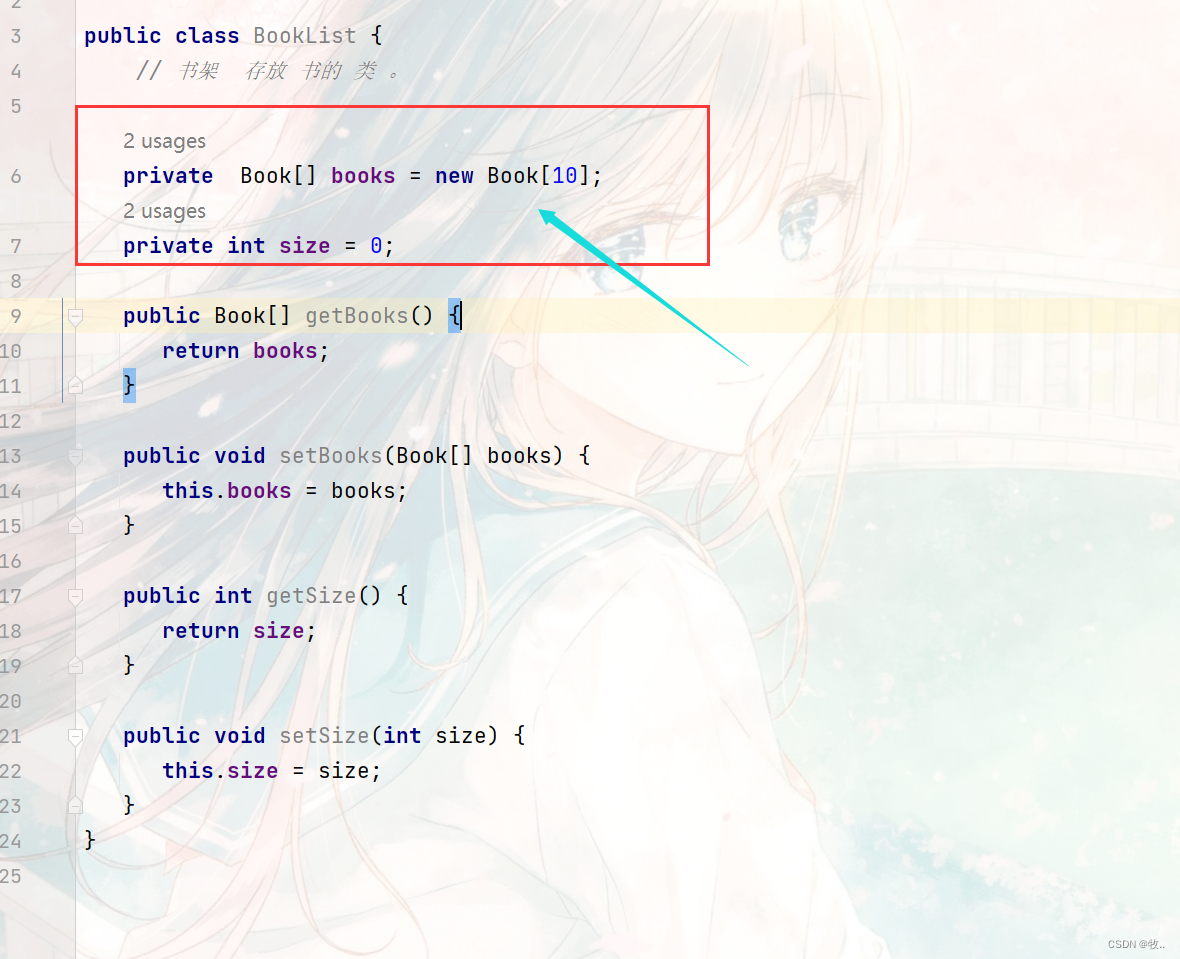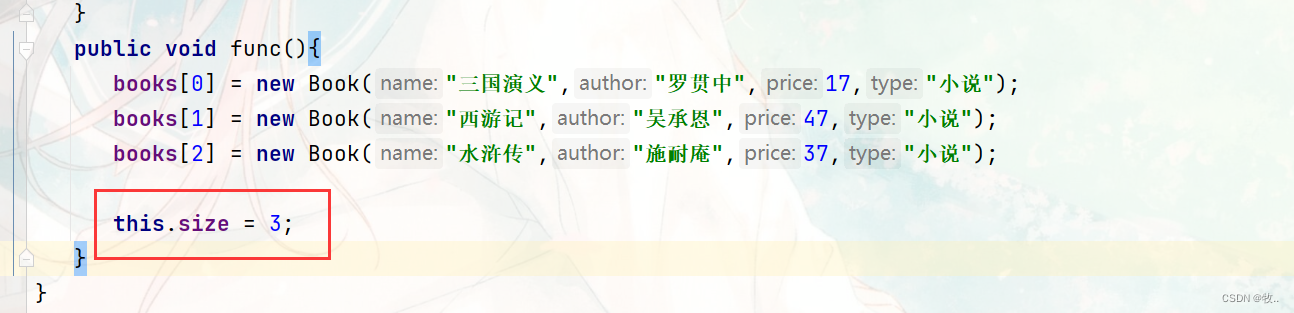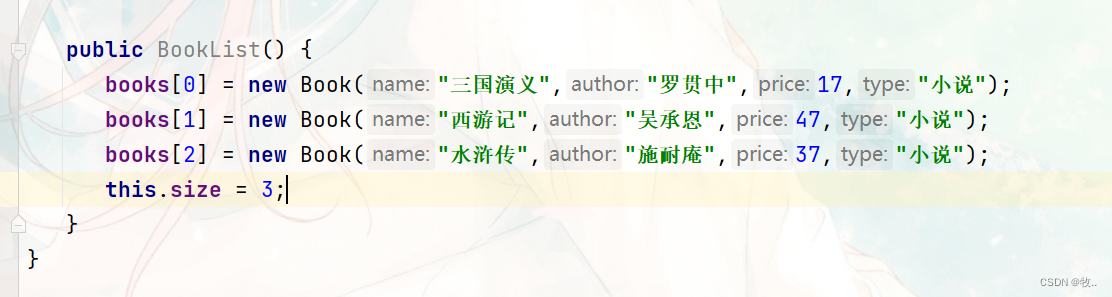``````public class BookList {
// 书架  存放 书的 类 。

private  Book[] books = new Book;
private int size = 0;

// 通过 getPos 获取当前 pos 位置的 书
public Book getPos(int pos) {
return books[pos];
}

// 设置 pos 位置 放一本书
public void setBook(int pos,Book book) {
this.books[pos] = book;
}

public int getSize() {
return size;
}

public void setSize(int size) {
this.size = size;
}
//   public void func(){
//      books = new Book("三国演义","罗贯中",17,"小说");
//      books = new Book("西游记","吴承恩",47,"小说");
//      books = new Book("水浒传","施耐庵",37,"小说");
//
//      this.size = 3;
//   }

public BookList() {
books = new Book("三国演义","罗贯中",17,"小说");
books = new Book("西游记","吴承恩",47,"小说");
books = new Book("水浒传","施耐庵",37,"小说");
this.size = 3;
}
}
``````

## 1.2 User 底下的 管理员类 和 用户类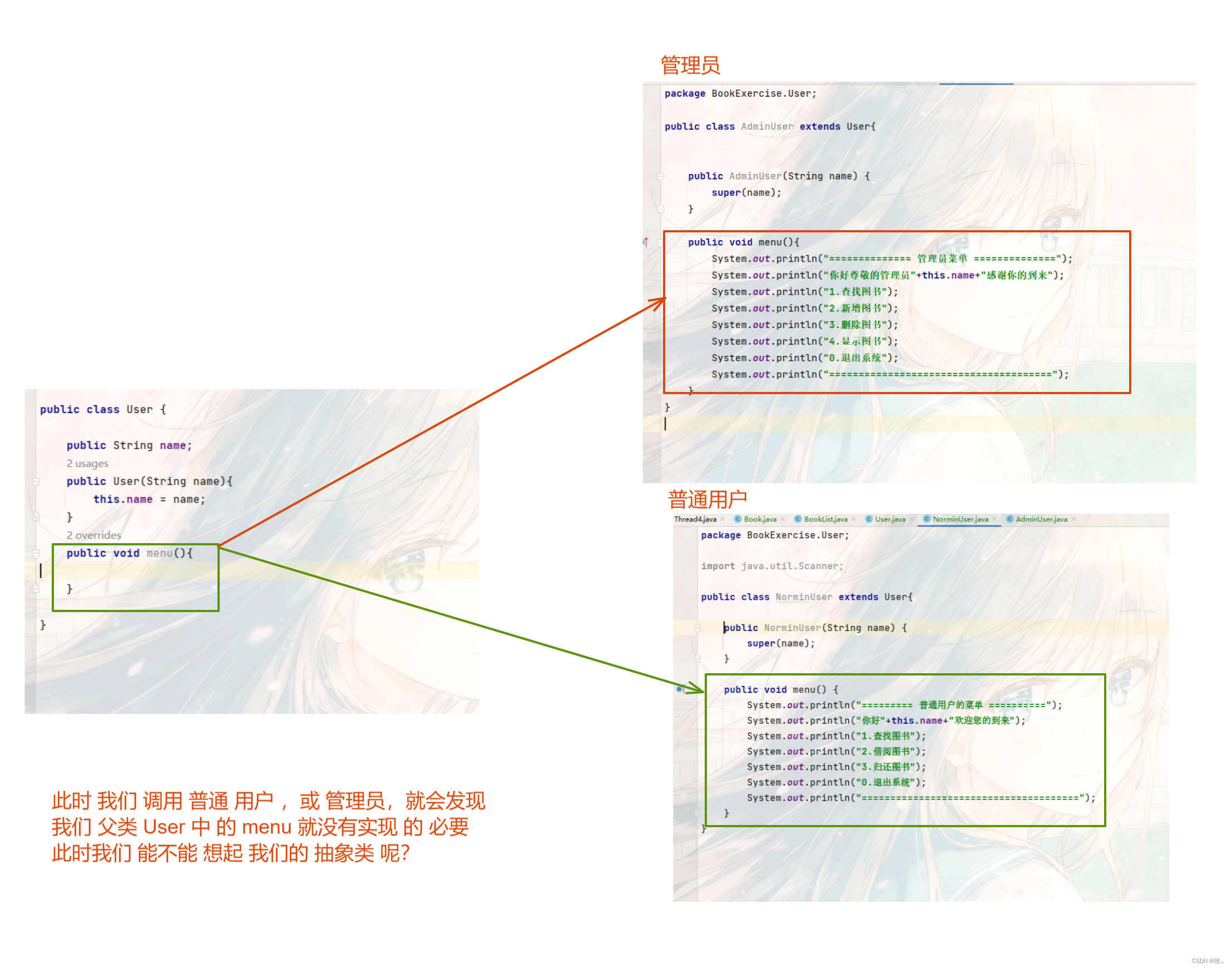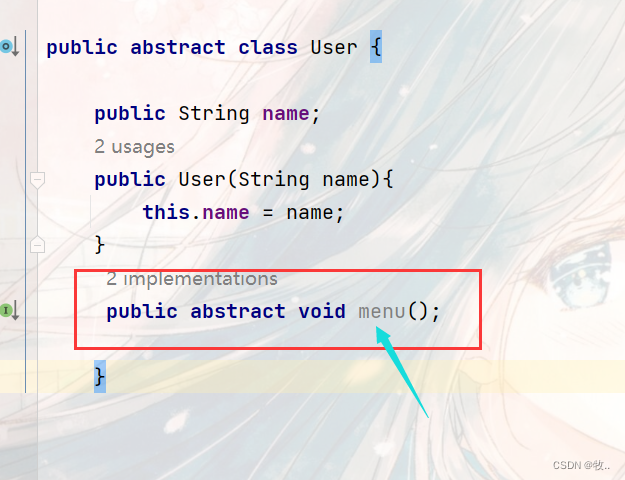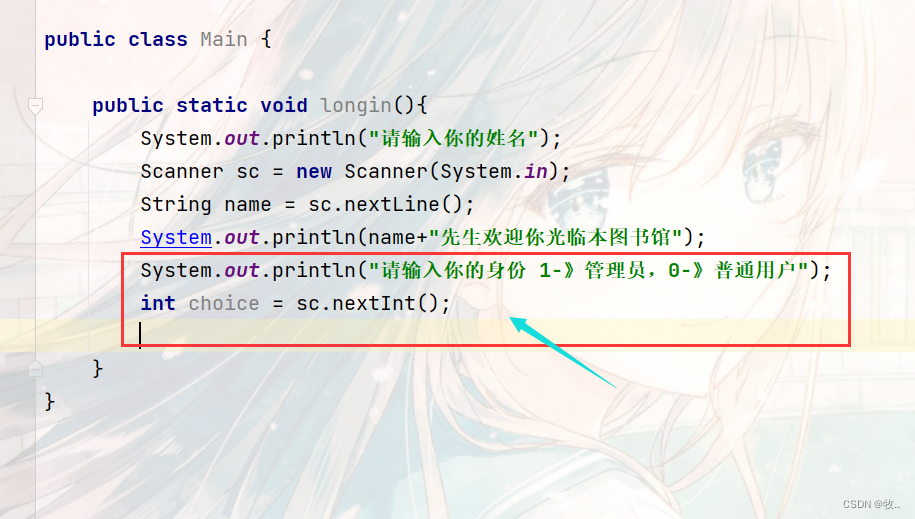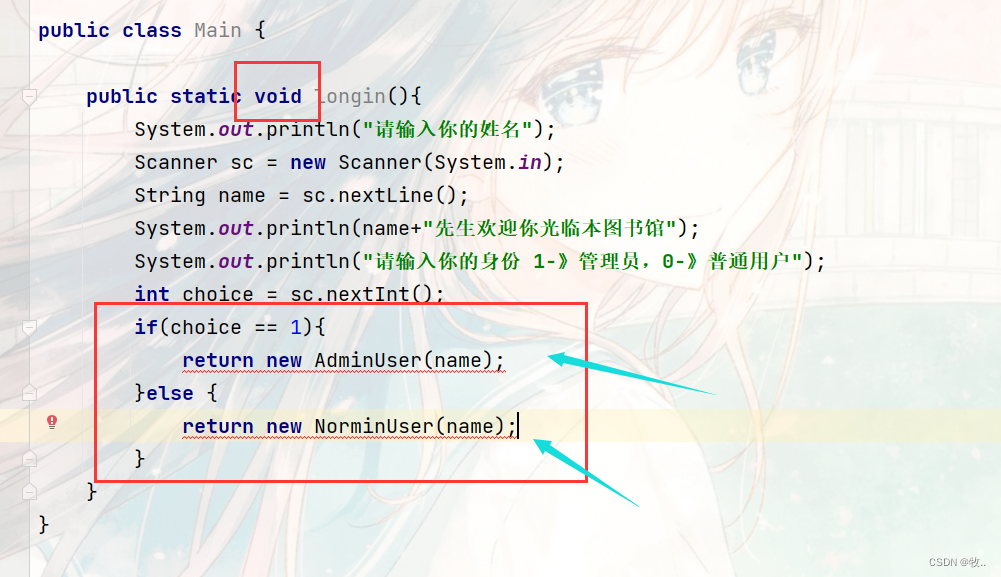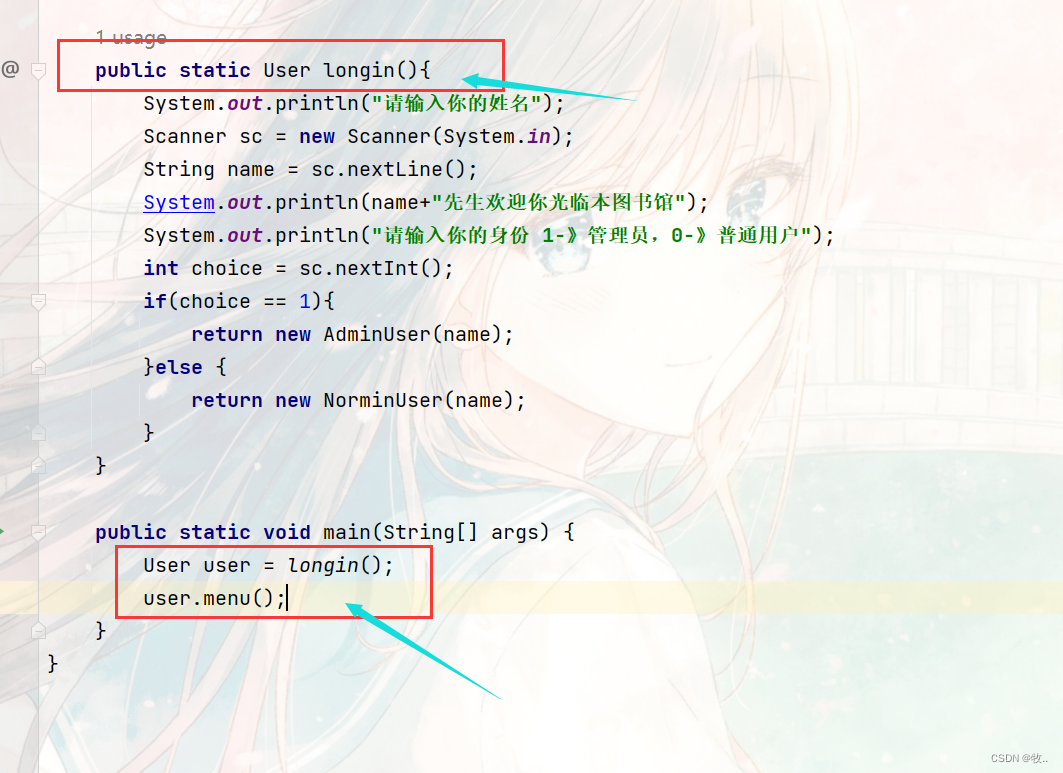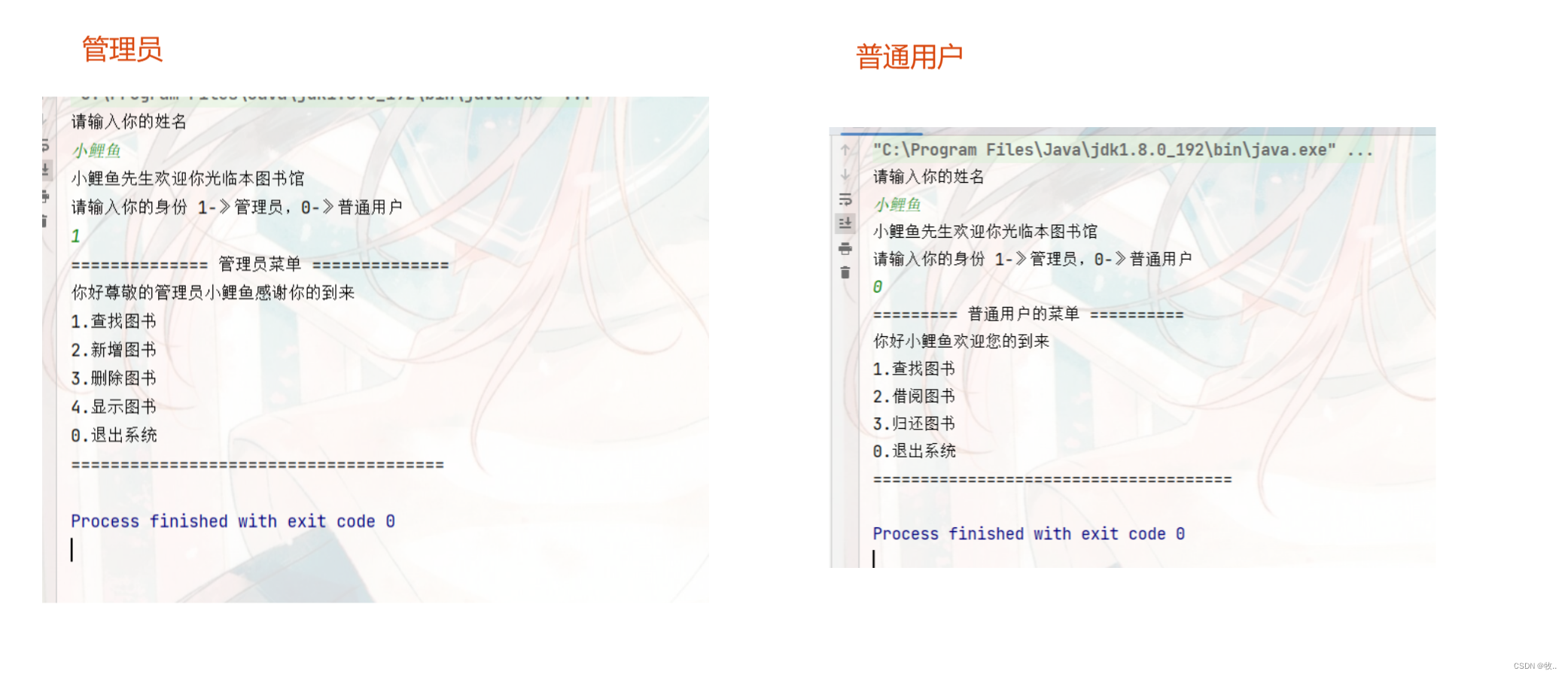## 1.3 管理员 and 用户 对应 方法串联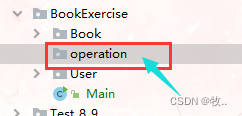ExitOperation : 退出系统 FindOpeation : 查找 图书 ReturnOperation ： 归还 图书 。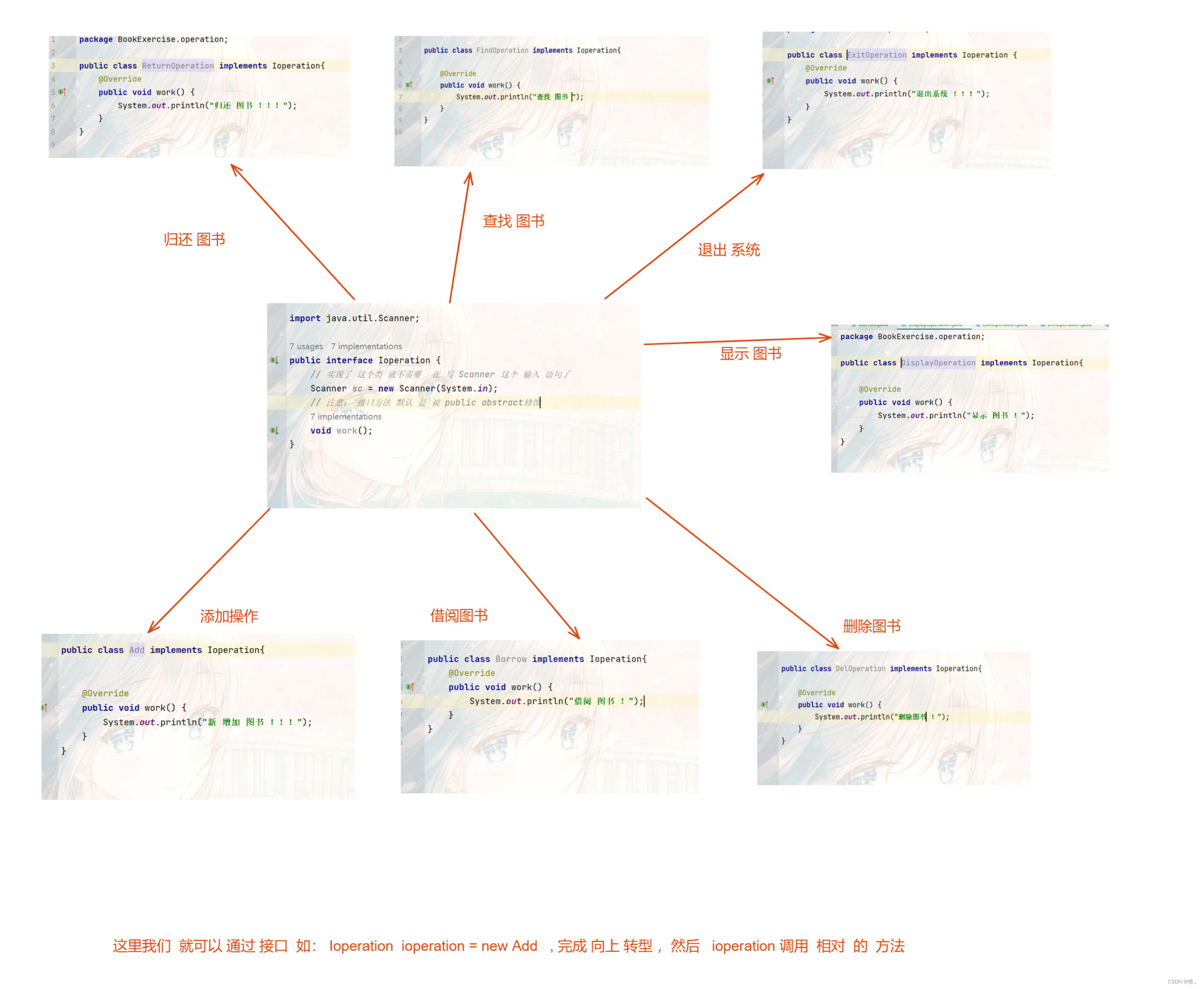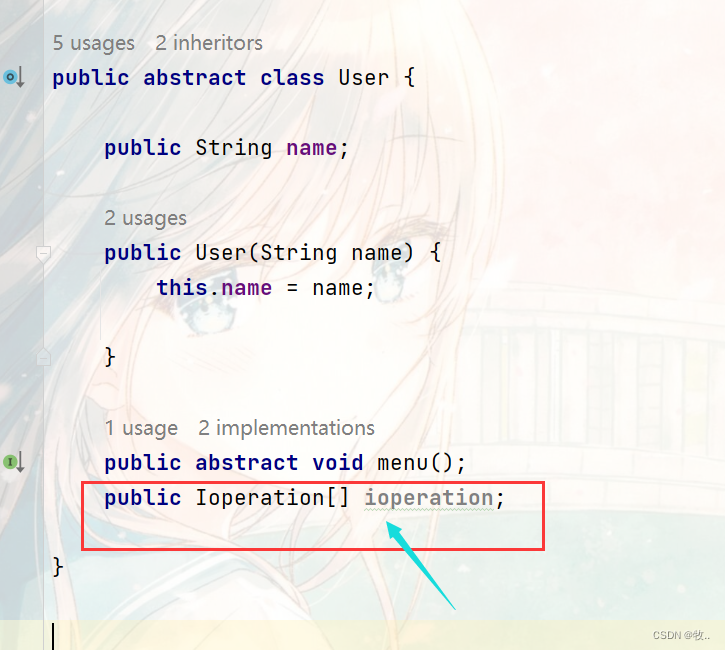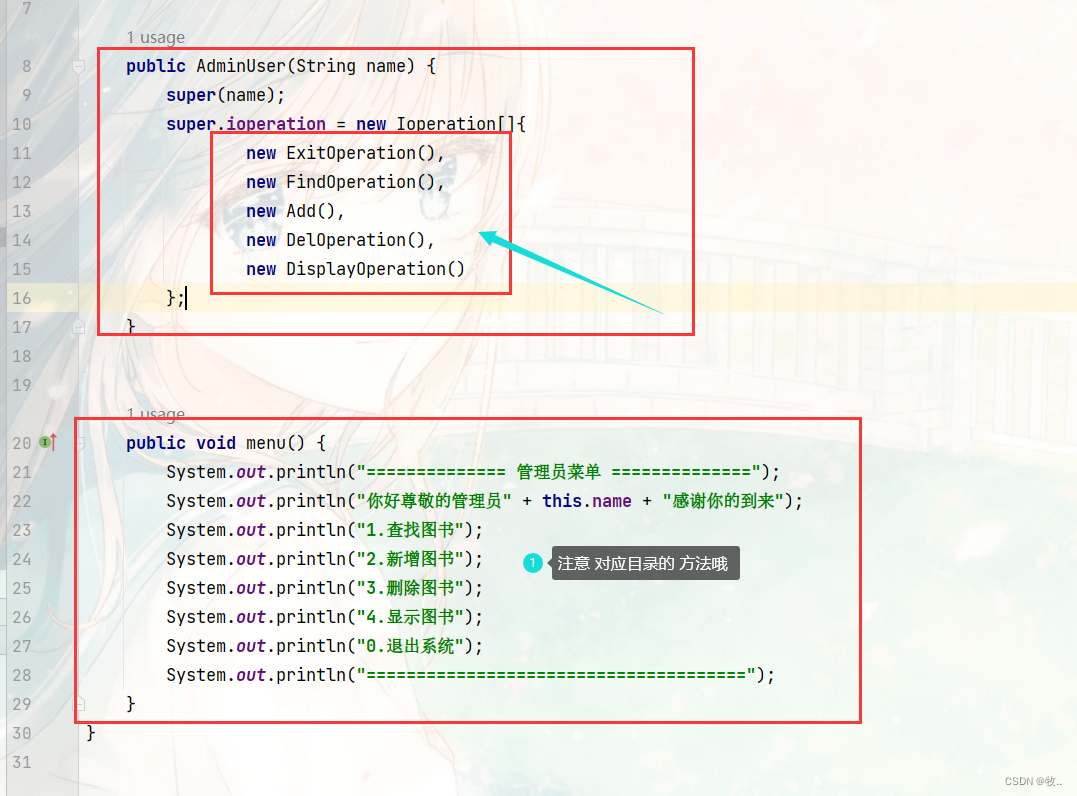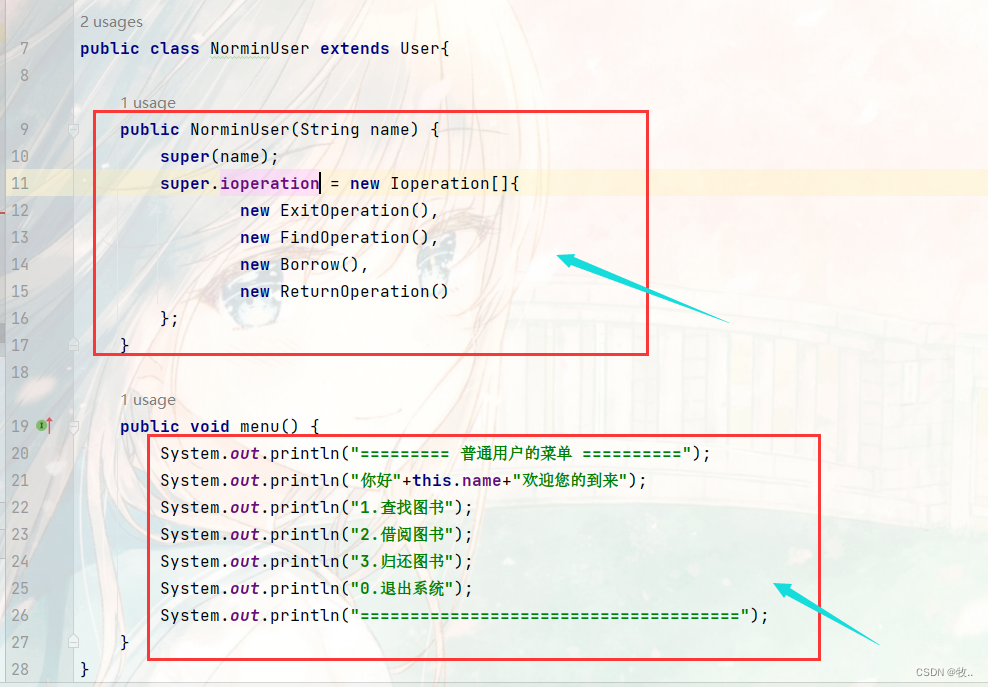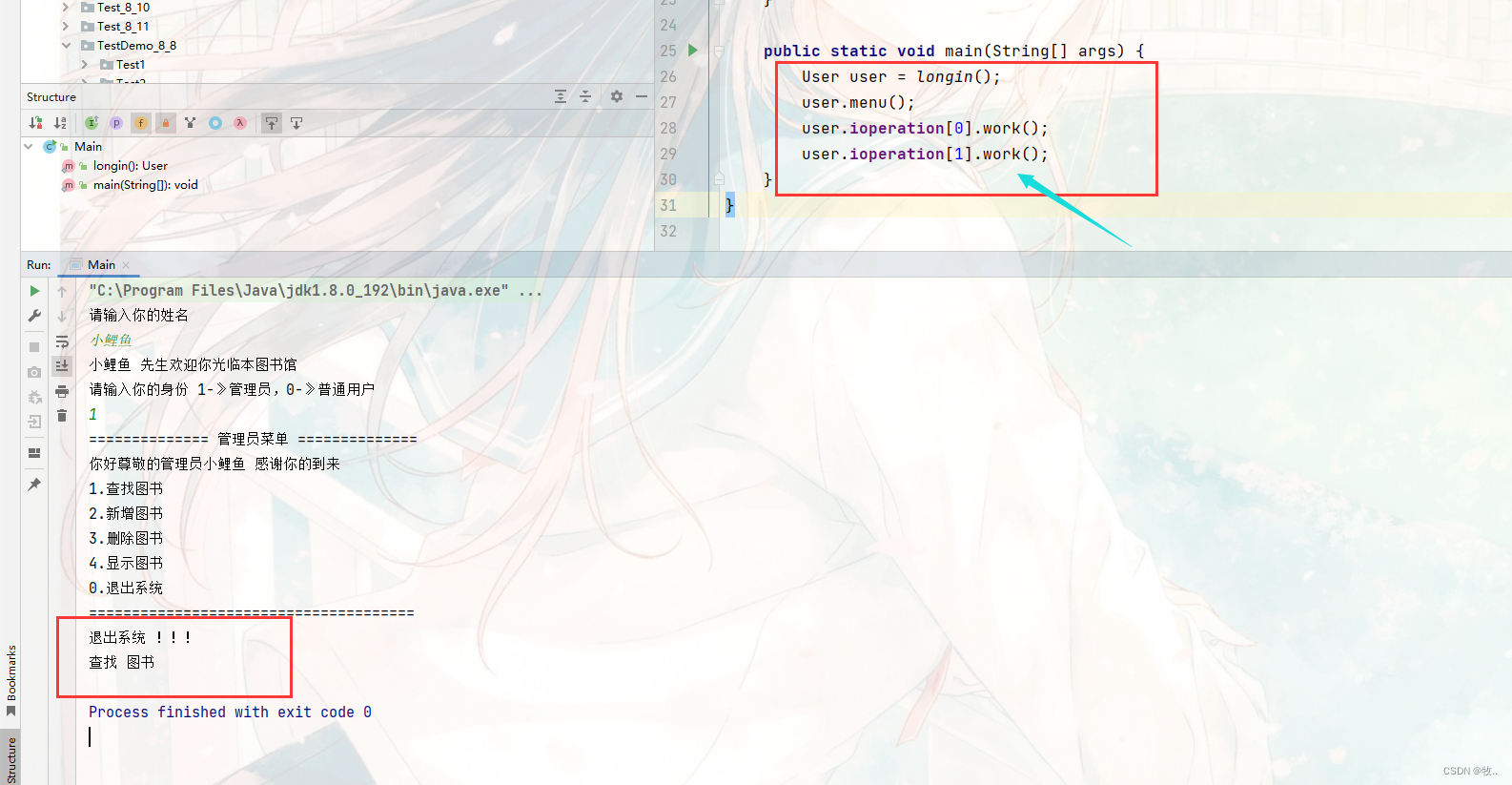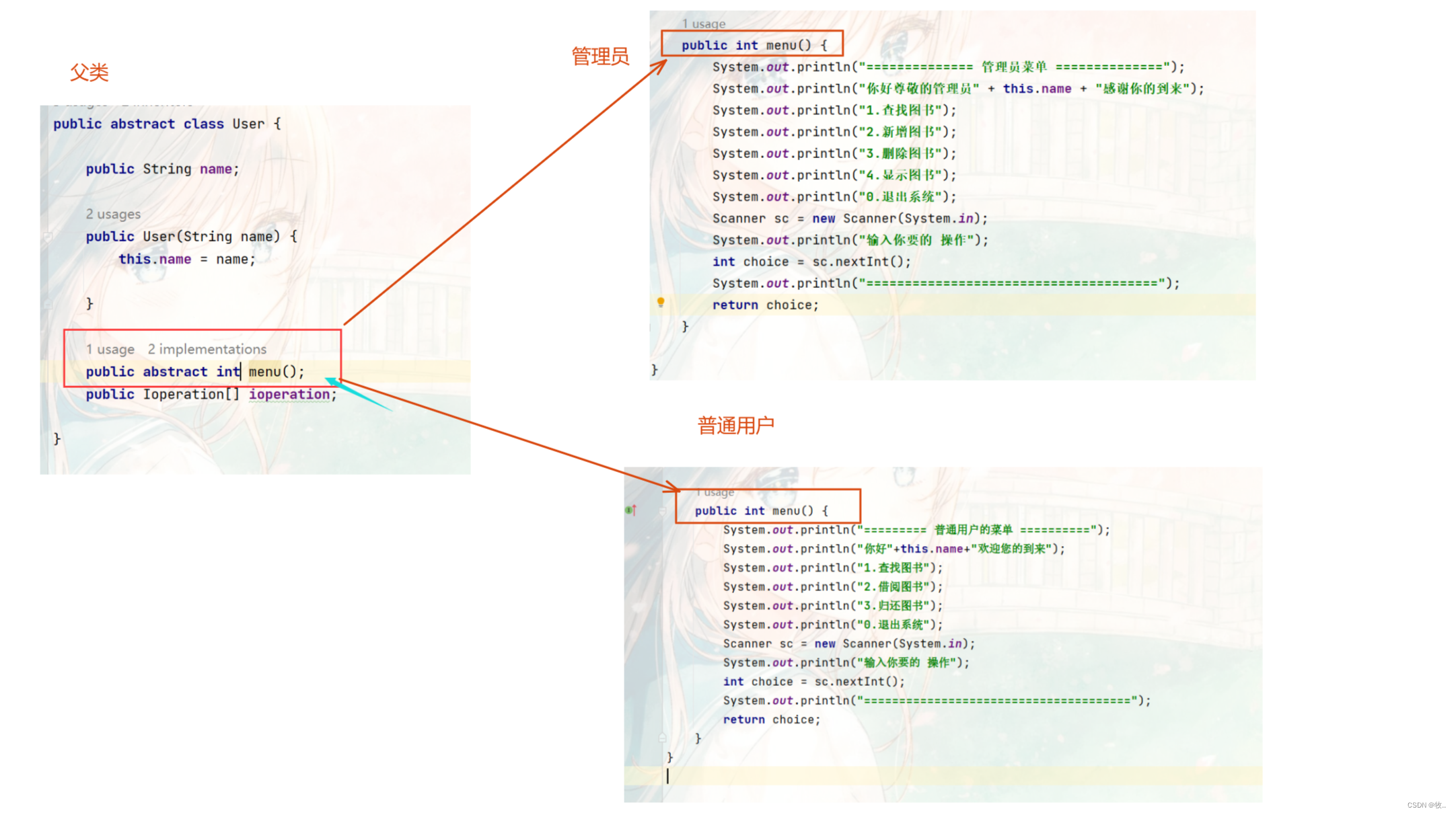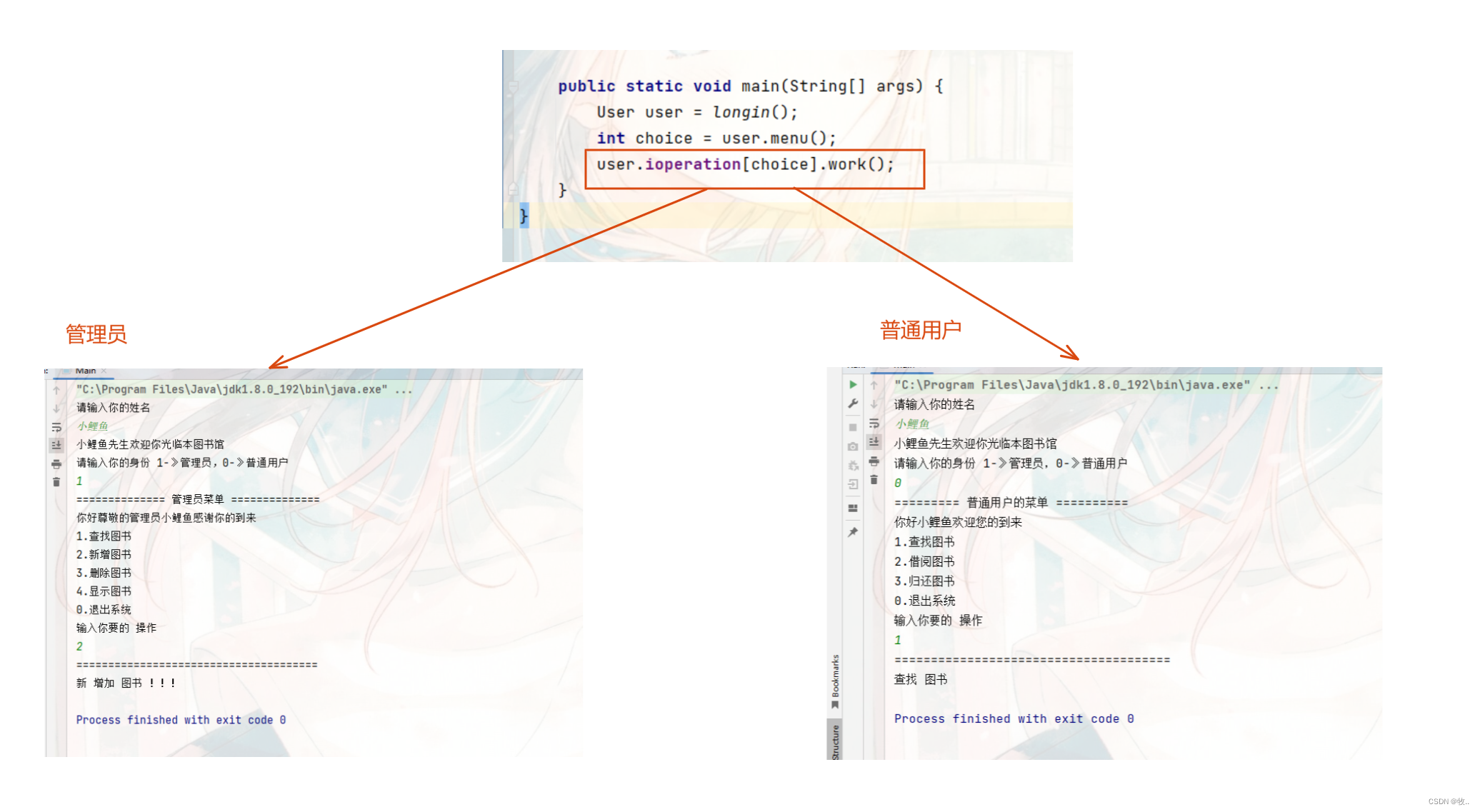# 2.管理员 and 用户 方法 实现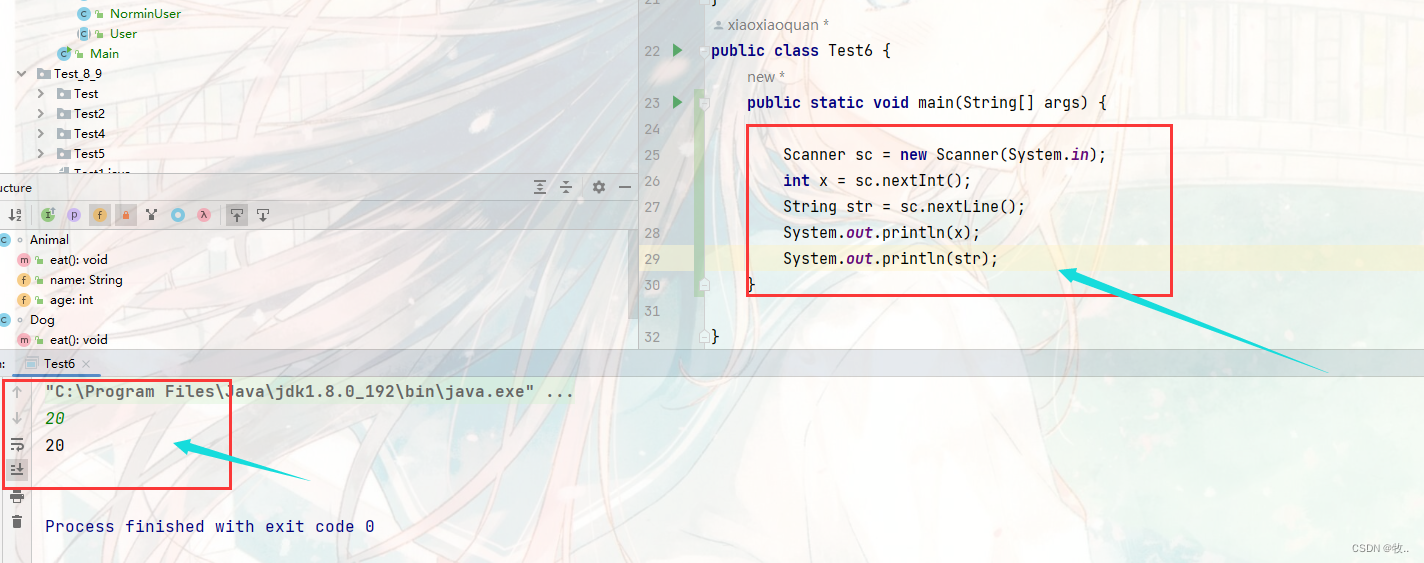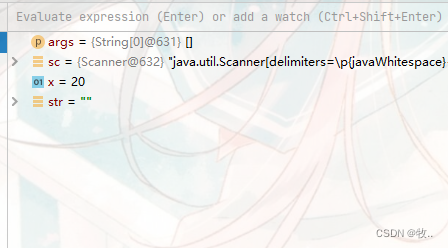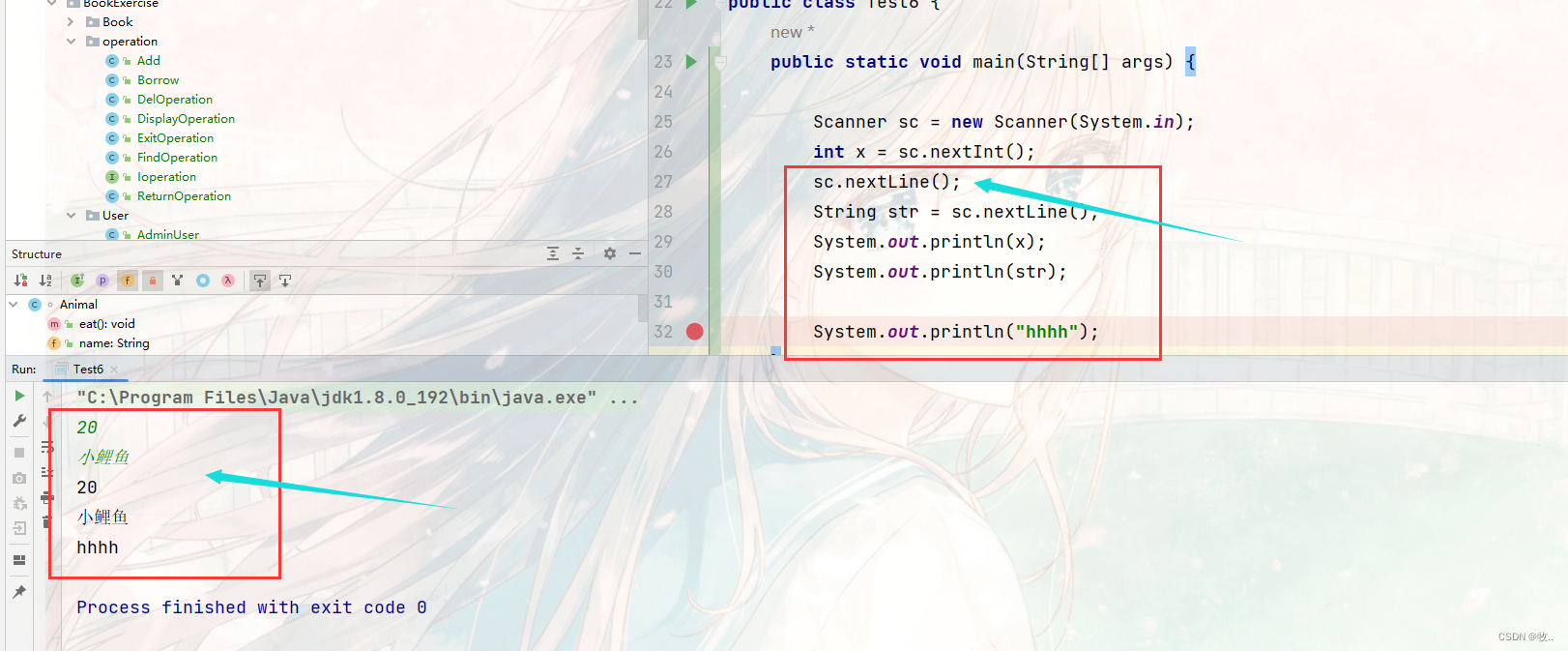``````public class Add implements Ioperation {
@Override
public void work(BookList bookList) {
// 添加 图书 。
System.out.println("新增 图书 ");
System.out.println("新增 图书 的 名字");
String name = sc.nextLine();
System.out.println("新增 图书的 作者");
String author = sc.nextLine();

System.out.println("新增 图书的 价格");
double price = sc.nextDouble();
// 吸收 赋值price 的 换行符
sc.nextLine();
System.out.println("新增 图书的 类型");
String type = sc.nextLine();
Book book = new Book(name, author, price, type);
// 判断是否 需要 扩容。
bookList.func();
// 拿到 当前 size 的 大小。
int size = bookList.getSize();
// 因为我们 的数组  封装起来了 ，提供 了 特有的 方法 让他添加 图书 ，这里就 通过这个方法
//完成我们的 图书 的添加
bookList.setBooks(size, book);
// 此操作为更新 图书
bookList.setSize(size + 1);
System.out.println("新增图书成功");
}
}
``````

## 2.2 Borrow - 借阅图书

``````public class Borrow implements Ioperation {
@Override
public void work(BookList bookList) {
// 借阅 图书 。
// 1.判断 该图书是否 存在 ，或 是否 被 借出 。
System.out.println("借阅 图书 区 ");
System.out.println("输入 想要 借阅 图书 的 名字");
String name = sc.nextLine();
int size = bookList.getSize();
for (int i = 0; i < size; i++) {
// 通过  我们 设计  方法 拿到 当前 i 位置 的 图书 ，
Book book = bookList.getBooks(i);
if (book.getName().equals(name)) {
// 此时 说明找到了 我们需要的 书 。
if (book.getisBorrowed()) {
System.out.println("该图书 已被借出  ");
} else {
book.setBorrowed(true);
System.out.println("借阅成功");
System.out.println(book);
}

}
}
System.out.println("本 图书 馆没有 此 书");

}
}

``````

## 2.3 DelOperation - 删除 图书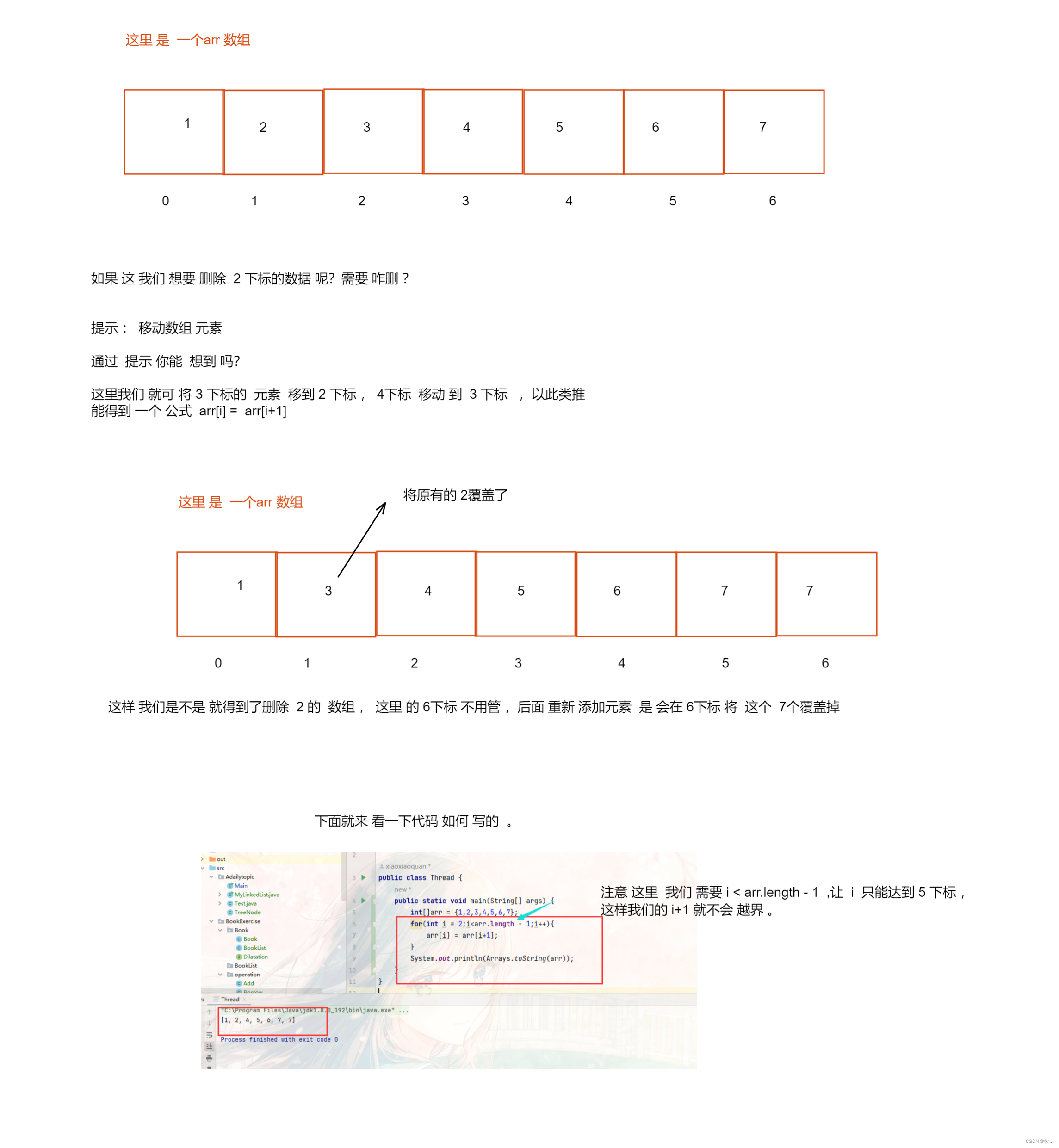``````public class DelOperation implements Ioperation {
@Override
public void work(BookList bookList) {
// 删除图书。
System.out.println("删除 图书 ");
System.out.println("输入 要删除 的 图书 名字");
String name = sc.nextLine();

// 顺序表 删除  挪位子。
int index = 0;
int size = bookList.getSize();
int i = 0;
for (i = 0; i < size; i++) {
// 通过 getBooks 拿到我们的 i 位置的 下标
Book book = bookList.getBooks(i);
if (book.getName().equals(name)) {
// 这里 不考虑 重复 的 书 ，只删除 第一本。
index = i;
//拿到 下标我们就 直接 退出 循环 即可
break;
}
}
// 如果  i 大于我们 的 图书 的 数量 ，那么 就说明 没有找到我们的 图书
if (i >= size) {
System.out.println("没有你要删除的 图书");
return;
}

for (int j = index; j < size - 1; j++) {
// 拿到 j + 1 本 书
Book book = bookList.getBooks(j + 1);
bookList.setBooks(j, book);
}
// 将 最后 一本 书 置为 空， 要不 会 出现 内存 泄露
bookList.setBooks(size - 1, null);
bookList.setSize(size - 1);
System.out.println("删除 成功");

}
}

``````

## 2.4 DisplayOperation - 显示 图书

``````public class DisplayOperation implements Ioperation {

public void work(BookList bookList) {
// 打印 图书  / 显示 图书

System.out.println("显示 全部 图书");
int size = bookList.getSize();
for (int i = 0; i < size; i++) {
Book book = bookList.getBooks(i);
System.out.println(book);
}
}
}
``````

## 2.5 ExitOperation - 退出 系统

``````public class ExitOperation implements Ioperation{
@Override
public void work(BookList bookList) {
System.out.println("退出 程序");
// 这里 exit(0) 表示正常 退出 系统
System.exit(0);
}
}
``````

## 2.6 FindOperation - 查找图书

``````public class FindOperation implements Ioperation {

@Override
public void work(BookList bookList) {
// 查找 图书

System.out.println("查找 图书 区 ");
System.out.println("输入 要查找 的 图书 ");
String name = sc.nextLine();
int size = bookList.getSize();
for (int i = 0; i < size; i++) {
Book book = bookList.getBooks(i);
if(book.getName().equals(name)){
System.out.println("找到此书,信息如下");
System.out.println(book);
return;
}
}
System.out.println("未 查找到 此 书");
}
}

``````

## 2.7 ReturnOperation - 归还图书

``````public class ReturnOperation implements Ioperation{
@Override
public void work(BookList bookList) {
// 归还 图书 ，
System.out.println("归还 图书 区");
System.out.println("请输入你要归还图书的名字");
String name = sc.nextLine();
int size = bookList.getSize();
for(int i = 0;i<size;i++){
Book book = bookList.getBooks(i);
if(book.getName().equals(name)){
// 将原本 借出 图书 的 true 置为  false
book.setBorrowed(false);
System.out.println("归还成功 ！！！");
System.out.println(book);
return;
}
}
System.out.println("此 书 不是 本 图书 馆 ，归还 失败");
}
}
``````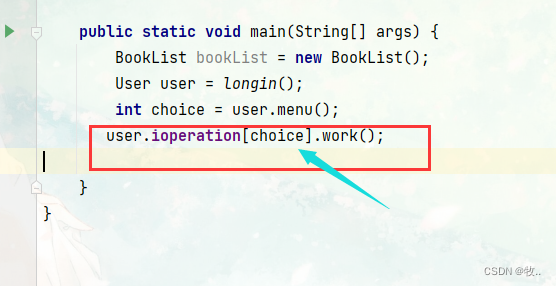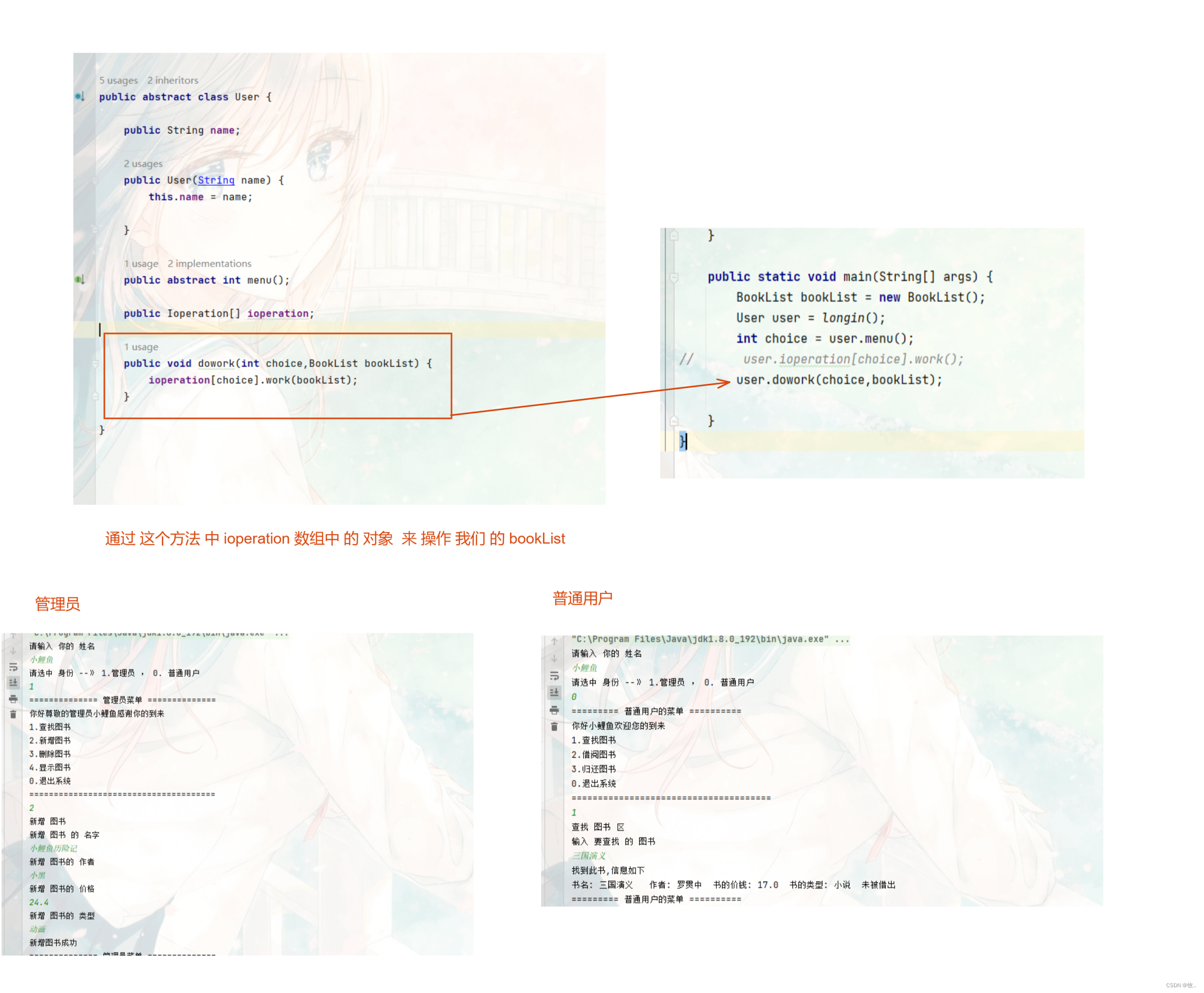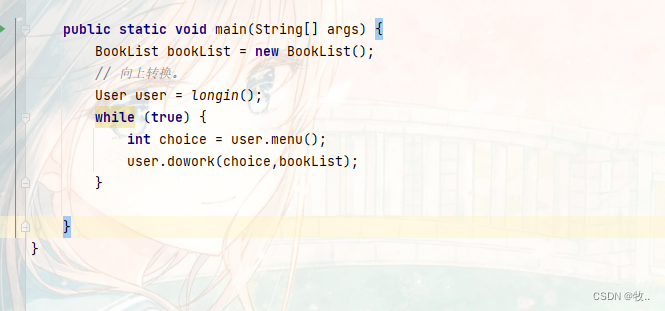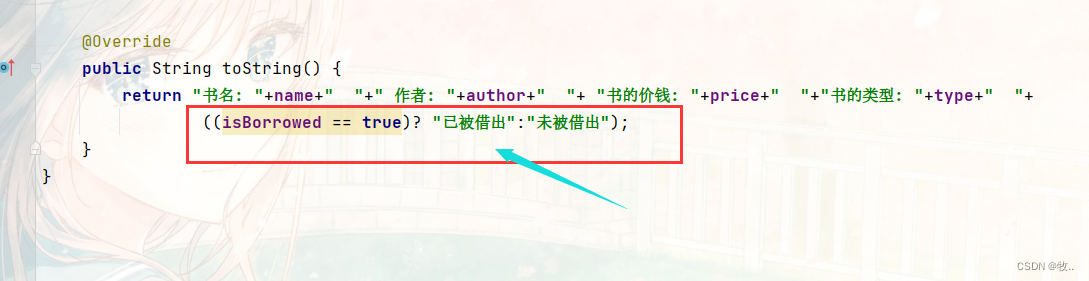### 网站公告